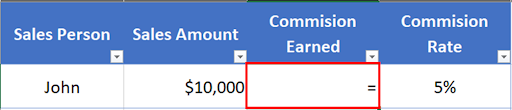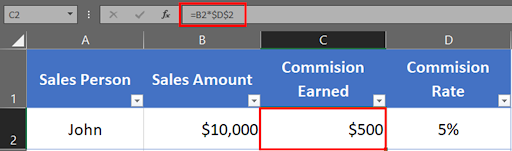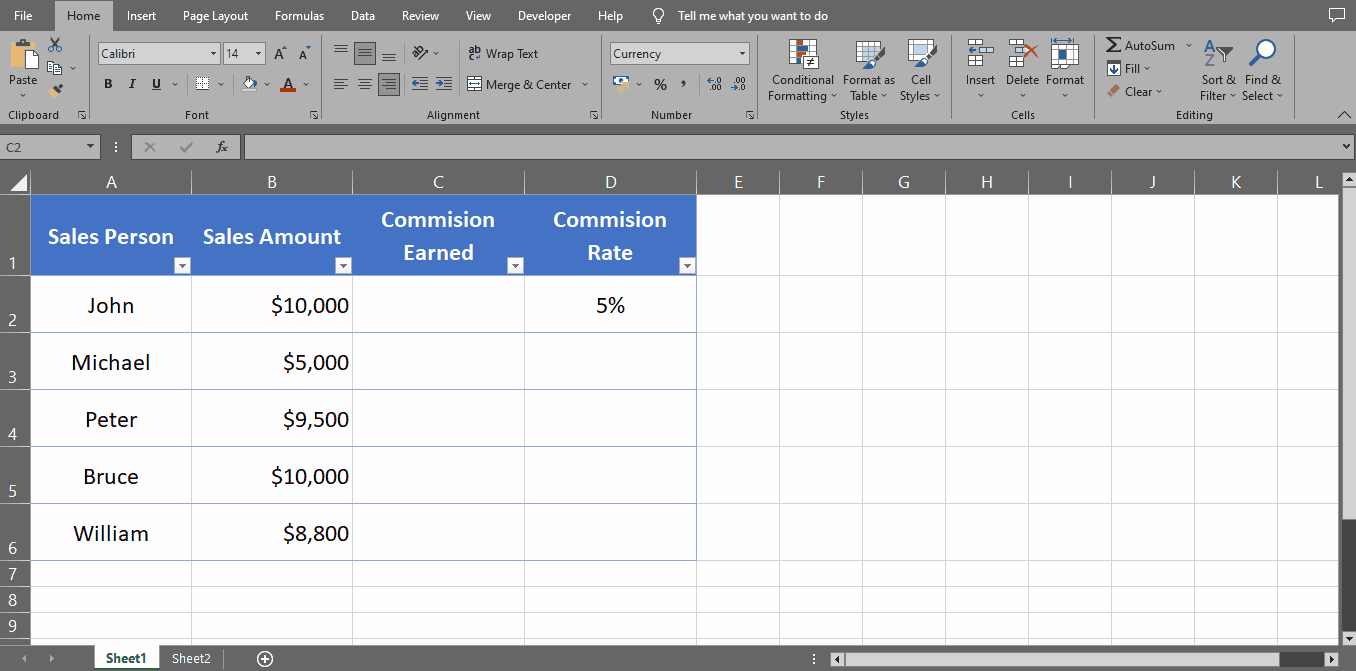# How to keep a formula constant in Excel

Let’s say you have a worksheet where you want to calculate the commission earned by each salesperson on their sales. You have the following data:

To calculate the commission earned by each salesperson, we will use the following formula:

= Sales amount * Commission rate

The formula multiplies the sales amount by the commission rate to calculate the commission earned.

Now, let’s say we want to keep the commission rate constant and not allow it to change if we copy and paste the formula into other cells. To do this, follow these steps:Excel has three types of cell references: relative, absolute, and mixed. Each type has a specific purpose and knowing how to use them is important for creating accurate formulas, proper formatting and calculating data.

Relative cell references: Relative cell references are the default type of reference used in Excel. When you copy a formula that contains a relative cell reference, the reference is automatically adjusted relative to the location of the formula.

Absolute cell references: Absolute cell references are used when you want to refer to a specific cell or range of cells in a formula, regardless of where the formula is copied.

Mixed cell references: Mixed cell references are a combination of relative and absolute cell references. You can use mixed cell references to keep a row or column constant while allowing the other part of the reference to change relative to the location of the formula.

### Step 1 – Select The Cell– Select the cell where you want to calculate the total & place equals to sign (=).

### Step 2 – Type The Formula– Select the cell containing the sales amount (in this case it’s cell B2).
– Type an asterisk sign (*).
– Type the dollar sign (\$) before the column letter and row number of the cell reference, like this: \$D\$2. This is called an absolute reference and will keep the cell reference constant when you copy and paste the formula. Alternatively, you can also use the F4 shortcut key after writing D2 and it will convert it into absolute cell reference.
– Press Enter to save the formula.

### Step 3 – Drag The Formula Downwards– With the help of the selection handle, drag the formula to the remaining cells down below.
– You will notice that the commission rate remains constant, but the sales amount reference changes to the cell below it.
– This is just one example of how to keep a formula constant in Excel using an absolute reference.